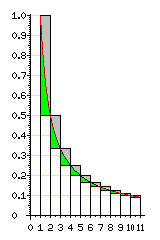[Mathematics] [Other] [Home]

# Euler's Constant

Euler's constant, sometimes called the Euler-Mascheroni constant, also known as gamma, has the value is .57721 56649 01532 86060 65120 90082... and is a less well known mathematical constant than pi or e, but it is still a very important one.

Euler's constant is defined as the limit, as n tends to infinity, of the sum of 1 + 1/2 + 1/3 + ... up to 1/n, minus the natural logarithm of n, normally written:

```                /     n                     \
|   /   ____       \          |
|  |    \      1    |         |
lim        |  |     >    ---   | - ln(n) |
n -> infinity  |  |    /___   i    |         |
|   \    i=1       /          |
\                           /
```

Since

```     _n
/
|   1
|  ---, dx = ln(n)
|   x
_/
1
```

that is, the integral from 1 to n of 1/x with respect to x is the natural logarithm of n, the following diagram illustrates what Euler's constant is in graphical form:Allowing the graph to continue on indefinitely to the right, the boxes shown which are divided into green and gray areas have a total overall area of 1. (Note that the vertical scale in the graph is exaggerated compared to the horizontal scale for clarity.) Euler's constant, .57721 56649... is the total of the areas of the gray parts of the boxes.

Because only scaling makes the shape of the graph of 1/x from 1 to 2 different from the graph of 1/x from 2 to 4, or the graph of 1/x from 4 to 8, the following diagram:illustrates a way in which it is possible to derive a formula for Euler's constant that would only involve calculating the natural logarithm of 2.

This formula is:

```                      infinity                    (i+1)
________    /              2                  \
\          /               ____                \
\        |                \        1     1     |
gamma = (1 - ln(2)) +   >       | (1 - ln(2)) -   >      --- - ---    |
/        |                /___      i    j     |
/_______   \                   i    2          /
i = 1     \             j = 2 +1            /
```

By superimposing the areas from 1 to 2, from 2 to 4, and 4 to 8 after adjusting them to fit, one sees that another series for Euler's constant, not involving any logarithms, is possible, as it is (1/2 - 1/3) + 2 * ((1/4 - 1/5) + (1/6 - 1/7)) + 3 * ((1/8 - 1/9) + (1/10 - 1/11) + (1/12 - 1/13) + (1/14 - 1/15)) + ..., or, in other words:

```         infinity          (i+1)
_________    /    2     - 1              \
\           /      ____                   \
\         |       \          (j-1)   1    |
gamma =   >        |  i *   >     (-1)     * ---   |
/         |       /___               j    |
/________   \          i                  /
i = 1      \    j = 2                  /
```

Euler's constant is more difficult to calculate than the square root of 2, e, or pi, and it is less well understood. Like any other number, it must be either rational, algebraic, or transcendental. But there is as yet no mathematical proof by means of which we can know for certain in which of these three classes it belongs.

[Mathematics] [Other] [Home]